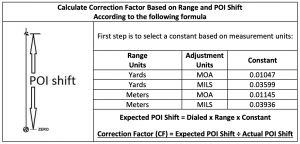## Tall Target Test Worksheet

The intent of this worksheet is to assist in calculating a scope correction factor (CF) based on shooting the tall target test at 100 yards. The point is to see if your scope is really giving you what you’re dialing for adjustment. If not, the correction factor is applied to raw ballistic calculations to make up for the error in scope adjustment.

### Procedure:

1) Set up a tall target at 100 yards with a vertical line (confirmed with plumb bob or level).

2) Place an aim point near the bottom of the vertical line and shoot a group to confirm zero.

3) Dial up (or hold) at least 30 MOA (or 10 MILS) of elevation and shoot another group.

4) Measure the distance between shot groups with a tape measure.

5) Use the formula below to calculate your scopes Correction Factor (CF).

6) Apply the Correction Factor to any raw ballistic solution to account for scope tracking error.### Example:

Suppose the range to target is 102 yards. You dial 30 MOA and get a POI shift of 29.8 inches. The formula will apply as follows:

1) Since you’re dealing with yards and MOA, select the Constant of 0.01047.

2) Next, calculate Expected POI Shift: Expected POI Shift = Dialed x Range x Constant Expected POI Shift = 30 MOA x 102 yards x 0.01047 = 32.04 inches.

3) Finally, calculate Correction Factor: CF = Expected POI Shift ÷ Actual POI Shift CF = 32.04 Inches ÷ 29.8 Inches CF = 1.075

4) Apply this correction factor to any raw ballistic prediction. So if the ballistics program calls for 30 MOA elevation for some shot, you should dial: 30 MOA x 1.075 = 32.25 MOA to actually get 30 MOA

The procedure is the same for any combination of units and range, just plug in your numbers and apply the proper Constant, calculate Expected POI Shift, and finally the Correction Factor.